Search by Topic

Resources tagged with Curious similar to Odds and Evens:

Filter by: Content type:
Age range:
Challenge level:

There are 68 results

Broad Topics > Habits of Mind > Curious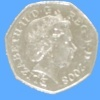Last One Standing

Age 14 to 16 Challenge Level:

Imagine a room full of people who keep flipping coins until they get a tail. Will anyone get six heads in a row?Which Spinners?

Age 14 to 18 Challenge Level:

Can you work out which spinners were used to generate the frequency charts?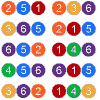Mathsland National Lottery

Age 14 to 16 Challenge Level:

Can you work out the probability of winning the Mathsland National Lottery? Try our simulator to test out your ideas.Odds and Evens Made Fair

Age 14 to 16 Challenge Level:

In this follow-up to the problem Odds and Evens, we invite you to analyse a probability situation in order to find the general solution for a fair game.Cosy Corner

Age 11 to 14 Challenge Level:

Six balls of various colours are randomly shaken into a trianglular arrangement. What is the probability of having at least one red in the corner?Two's Company

Age 11 to 14 Challenge Level:

7 balls are shaken in a container. You win if the two blue balls touch. What is the probability of winning?Same Number!

Age 14 to 16 Challenge Level:

If everyone in your class picked a number from 1 to 225, do you think any two people would pick the same number?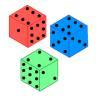Non-transitive Dice

Age 11 to 14 Challenge Level:

Alison and Charlie are playing a game. Charlie wants to go first so Alison lets him. Was that such a good idea?Reversals

Age 11 to 14 Challenge Level:

Where should you start, if you want to finish back where you started?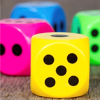Dicey Operations

Age 11 to 14 Challenge Level:

Who said that adding, subtracting, multiplying and dividing couldn't be fun?Special Numbers

Age 11 to 14 Challenge Level:

My two digit number is special because adding the sum of its digits to the product of its digits gives me my original number. What could my number be?Which Solids Can We Make?

Age 11 to 14 Challenge Level:

Interior angles can help us to work out which polygons will tessellate. Can we use similar ideas to predict which polygons combine to create semi-regular solids?A Little Light Thinking

Age 14 to 16 Challenge Level:

Here is a machine with four coloured lights. Can you make two lights switch on at once? Three lights? All four lights?Charlie's Delightful Machine

Age 11 to 16 Challenge Level:

Here is a machine with four coloured lights. Can you develop a strategy to work out the rules controlling each light?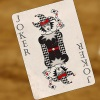Statement Snap

Age 7 to 14 Challenge Level:

You'll need to know your number properties to win a game of Statement Snap...Satisfying Statements

Age 11 to 14 Challenge Level:

Can you find any two-digit numbers that satisfy all of these statements?Stars

Age 11 to 14 Challenge Level:

Can you find a relationship between the number of dots on the circle and the number of steps that will ensure that all points are hit?How Much Can We Spend?

Age 11 to 14 Challenge Level:

A country has decided to have just two different coins, 3z and 5z coins. Which totals can be made? Is there a largest total that cannot be made? How do you know?A Chance to Win?

Age 11 to 14 Challenge Level:

Imagine you were given the chance to win some money... and imagine you had nothing to lose...Number Pyramids

Age 11 to 14 Challenge Level:

Try entering different sets of numbers in the number pyramids. How does the total at the top change?Unequal Averages

Age 11 to 14 Challenge Level:

Play around with sets of five numbers and see what you can discover about different types of average...On the Edge

Age 11 to 14 Challenge Level:

If you move the tiles around, can you make squares with different coloured edges?Nice or Nasty

Age 7 to 14 Challenge Level:

There are nasty versions of this dice game but we'll start with the nice ones...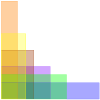Perimeter Possibilities

Age 11 to 14 Challenge Level:

I'm thinking of a rectangle with an area of 24. What could its perimeter be?Shifting Times Tables

Age 11 to 14 Challenge Level:

Can you find a way to identify times tables after they have been shifted up or down?Summing Consecutive Numbers

Age 11 to 14 Challenge Level:

15 = 7 + 8 and 10 = 1 + 2 + 3 + 4. Can you say which numbers can be expressed as the sum of two or more consecutive integers?Semi-regular Tessellations

Age 11 to 16 Challenge Level:

Semi-regular tessellations combine two or more different regular polygons to fill the plane. Can you find all the semi-regular tessellations?Elevenses

Age 11 to 14 Challenge Level:

How many pairs of numbers can you find that add up to a multiple of 11? Do you notice anything interesting about your results?How Old Am I?

Age 14 to 16 Challenge Level:

In 15 years' time my age will be the square of my age 15 years ago. Can you work out my age, and when I had other special birthdays?Litov's Mean Value Theorem

Age 11 to 14 Challenge Level:

Start with two numbers and generate a sequence where the next number is the mean of the last two numbers...Searching for Mean(ing)

Age 11 to 14 Challenge Level:

Imagine you have a large supply of 3kg and 8kg weights. How many of each weight would you need for the average (mean) of the weights to be 6kg? What other averages could you have?Estimating Time

Age 11 to 14 Challenge Level:

How well can you estimate 10 seconds? Investigate with our timing tool.Who's the Best?

Age 11 to 14 Challenge Level:

Which countries have the most naturally athletic populations?Multiplication Arithmagons

Age 14 to 16 Challenge Level:

Can you find the values at the vertices when you know the values on the edges of these multiplication arithmagons?Cola Can

Age 11 to 14 Challenge Level:

An aluminium can contains 330 ml of cola. If the can's diameter is 6 cm what is the can's height?What Numbers Can We Make?

Age 11 to 14 Challenge Level:

Imagine we have four bags containing a large number of 1s, 4s, 7s and 10s. What numbers can we make?Arithmagons

Age 14 to 16 Challenge Level:

Can you find the values at the vertices when you know the values on the edges?Sending a Parcel

Age 11 to 14 Challenge Level:

What is the greatest volume you can get for a rectangular (cuboid) parcel if the maximum combined length and girth are 2 metres?Blue and White

Age 11 to 14 Challenge Level:

Identical squares of side one unit contain some circles shaded blue. In which of the four examples is the shaded area greatest?Can They Be Equal?

Age 11 to 14 Challenge Level:

Can you find rectangles where the value of the area is the same as the value of the perimeter?Largest Product

Age 11 to 14 Challenge Level:

Which set of numbers that add to 10 have the largest product?More Number Pyramids

Age 11 to 14 Challenge Level:

When number pyramids have a sequence on the bottom layer, some interesting patterns emerge...Think of Two Numbers

Age 11 to 14 Challenge Level:

Think of two whole numbers under 10, and follow the steps. I can work out both your numbers very quickly. How?Age 11 to 14 Challenge Level:

Think of a number and follow my instructions. Tell me your answer, and I'll tell you what you started with! Can you explain how I know?Hexy-metry

Age 14 to 16 Challenge Level:

A hexagon, with sides alternately a and b units in length, is inscribed in a circle. How big is the radius of the circle?What Numbers Can We Make Now?

Age 11 to 14 Challenge Level:

Imagine we have four bags containing numbers from a sequence. What numbers can we make now?Marbles in a Box

Age 11 to 16 Challenge Level:

How many winning lines can you make in a three-dimensional version of noughts and crosses?Data Matching

Age 14 to 18 Challenge Level:

Use your skill and judgement to match the sets of random data.Opposite Vertices

Age 11 to 14 Challenge Level:

Can you recreate squares and rhombuses if you are only given a side or a diagonal?Square Coordinates

Age 11 to 14 Challenge Level:

A tilted square is a square with no horizontal sides. Can you devise a general instruction for the construction of a square when you are given just one of its sides?